Back-end Engineering Articles

I write and talk about backend stuff like Ruby, Ruby On Rails, Databases, Testing, Architecture / Infrastructure / System Design, Cloud, DevOps, Backgroud Jobs, and more...

@daniel_moralesp

2019-07-12

## Introduction to Logarithms in Data Structures

In the blog post about Big O Notation, we talked about three basic notations like O(1) - Constant Time, O(n) Linear Time, and O(n**2) Quadratic Time, to describe the time and space complexity of a given algorithm.

However, at the end of the article, we talked about other notations that help us measure times and space complexity but for different algorithms. Actually, I shared my personal cheatsheet about Big O Notation. Here you can see the image again: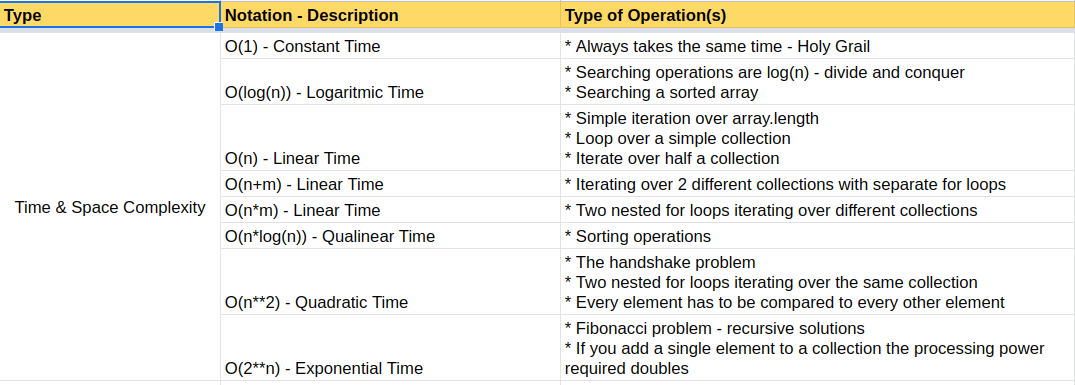As you can see, we have 2 other notations related to Logarithms (please don't confuse Logarithm with Algorithm). That notation is described as log(n). Now we're going to focus just on O(log(n)) Logarithmic Time

# O(log(n)) - Logarithmic Time

This notation can be pretty scary initially, but I'm going to do my best to explain at a high level what this means.

The first thing we have to check is the notation graph, and it looks like this.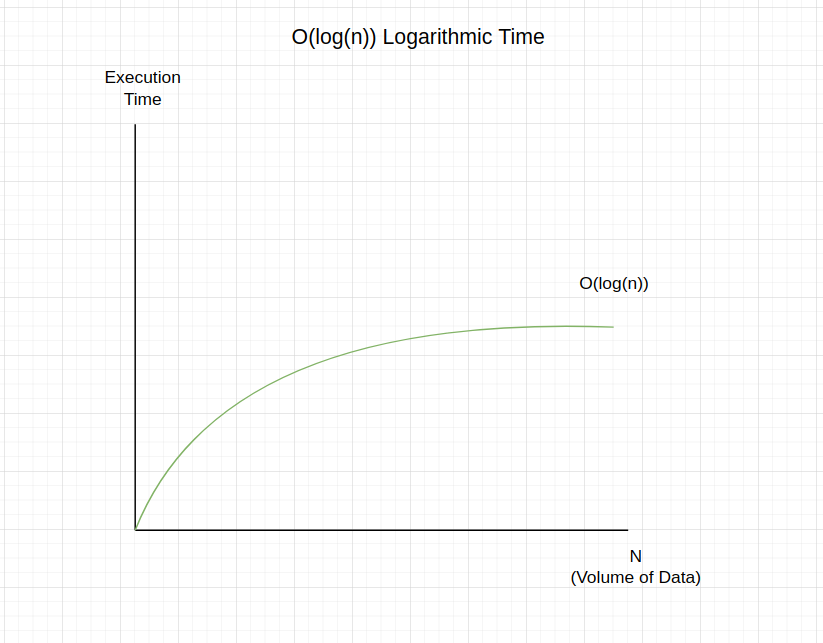Follow the blog post mentioned above. You can see that a problem solved using logarithmic time complexity is a good one because when we increase N (or volume of data in the array), the execution time tends to stabilize even if we have a bunch of new data. That's the magic behind the logarithmic solutions.

# Understanding Logarithms

I think the easiest way to explain this concept is as follows.

What are the results of the following operations in Ruby? (open your IRB console and test by yourself)

```➜  ~ irb
2.6.8 :001 > 2**1
=> 2
2.6.8 :002 > 2**2
=> 4
2.6.8 :003 > 2**3
=> 8
2.6.8 :004 > 2**4
=> 16
2.6.8 :005 > 2**5
=> 32
2.6.8 :006 > 2**6
=> 64
2.6.8 :007 > 2**7
=> 128
2.6.8 :008 > 2**8
=> 256 ```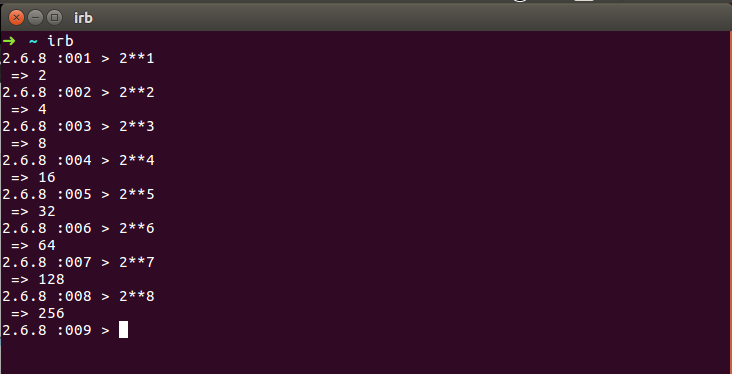Let's figure out what's happening here:

• * All the operations were made by calculating the 2 to the power of 1, 2, 3, 4, etc.
• * All the exponentials change just by 1. So, we started with 1, then 2, then 3, and so on. So the change is just by 1
• * If you see the results of each operation, we have other math facts.
•   * First result == 2
•   * Second result == 4 (which is the double of the last result)
•   * Third result == 8 (which is the double of the last result)
•   * Fourth result == 16 (which is the double of the last result)
•   * Fifth result == 32 (which is the double of the last result)
•   * Sixth result == 64 (which is the double of the last result)

• I think you got it!

• The conclusion is that we're changing the exponential just by 1, but the result is double the last operation.

This is why the graph we saw before tends to stabilize even if we have significant numbers because the exponent change just by 1; let's see the diagram explaining this effect.The latest graph is imperfect in the axis ticks, but it gives you the main idea.

# Logarithmic formula

Once we understand the result of the logarithm in a graph is easier to see the math formula.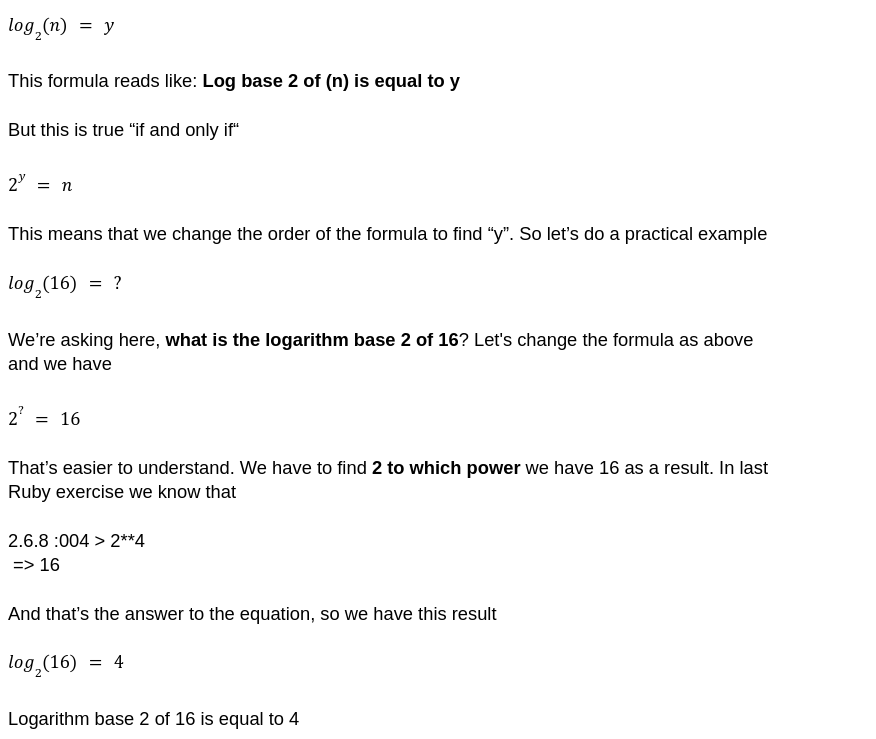Note: in computer science, to check the time and space complexity with this notation, we'll constantly be using logarithms base 2. (not base 10 or others)

If you want to experiment with other values, you can always do it yourself or look for calculators on the internet, like this: https://www.omnicalculator.com/math/log-2.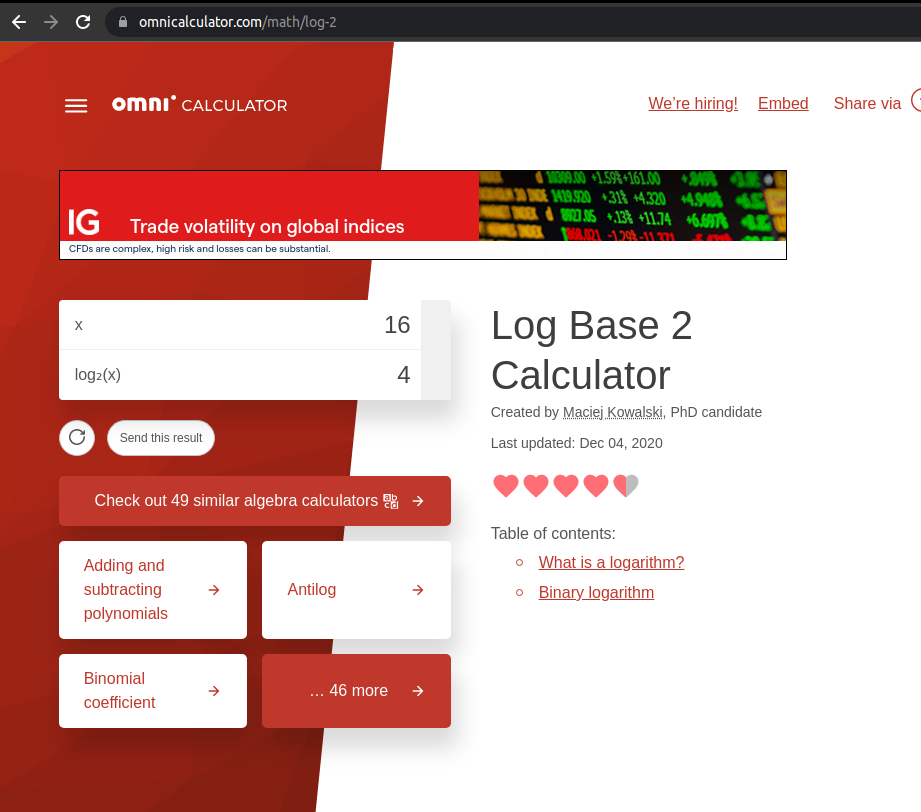# How can we translate this formula to code?

Now the idea is to see what kind of coding problems are logarithmic. Let's imagine an array of 100 elements, from 1 to 100. In that case, the formula is expressed by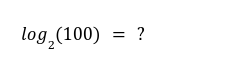If we do this operation using the calculator we shared before, the result should be 6.644. This means that in just 6.644 iterations, we'll resolve the coding problem!

But what coding problem?

A common coding problem that has this kind of behavior is searching operations. For instance, we have 100 array elements in ascending order, and we are asked to find the number 22 in the array. One way to solve this is called "divide and conquer," so we cut the array to half and choose the left side of that split. So we end with the first 50 elements. Then we repeat the process and cut it in half, ending up with the first 25 elements, and inside that array, we'll find the number 22.

Of course, we have different ways to solve this coding problem. Still, here we're talking about searching operations (like "divide and conquer") as a solution that meets the requirements to be logarithmic.

Later in new blog posts, we'll be solving these kinds of coding problems. But, for now, I think we have enough theory to understand the logarithmic notation.

I hope you learned a lot.